# How to Python: The Basics

Data Types, Comments, Errors, Variables, Arithmetic Operators and Inputs

When I wrote my last tutorial post, there was one person who I was confident was going to go through the steps of the blog post and put my lesson to the test. That is why it was no surprise to me that, when I had my weekly video chat with my dad, he came prepared with questions about the blog post. That is exactly the type of person my dad is. The first question he had for me also happened to be the most natural: “The post was very easy to follow and I have both Jupyter and Anaconda installed but HOW DO I CODE?”.

There are many in-depth resources that you can find on the web to begin writing code in Python but I will be writing my own very simple guide to help you start. This guide will cover the very basics of coding in Python 3 and my goal is to get you, the reader, writing code and playing with your code as quickly as possible.

I will be using Jupyter Notebook as my IDE. My last blog post briefly covered what an IDE is and how to install Jupyter Notebook. For reference, here is the link: https://medium.com/@johnkundycki/start-coding-now-python-for-data-science-4270525b7198

Without further ado, let us get into it.

# Python

Before we write any code, let me highlight two important facts about Python. First, Python is an Object Oriented Language. What this means is that everything is treated as what is called an object. You can ask Python for help on objects by typing help(<object>) to get information on the object or dir(<object>) to show the object’s methods. As we continue through the lesson, I will no longer include the placeholder <object> in the methods I mention. You’ll see what I mean shortly.

Second, Python is case sensitive, which means that var and VAR are not the same thing (same goes for x and X and john and John).

# Lesson

For the lesson, I will provide you with the code which you can copy and paste into your IDE to run it. Following the code will be an image of what the code should look like and what should happen when you run it.

Data Types
Alright, let’s start coding. In the last lesson, I showcased the most well-known code snippet to exist: print(“Hello World”). What is a code snippet you ask? A code snippet is a small region of reusable code and every image containing code in this post is a code snippet.

Let’s give it a shot! Type the code in your cell and hit Shift+Enter to run the cell.

`print("Hello World!")`

It should look as follows: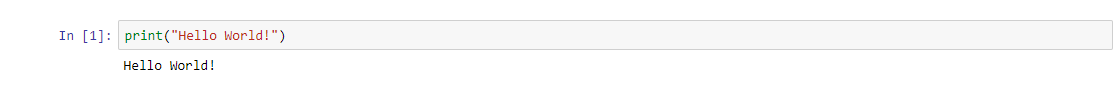The “In :” is unique to Jupyter Notebook and and allows the user to see the order in which they ran different cells.

In this snippet we are asking our machines to print (which is to return) a data type called a string (str) by employing the print() method. In laymen’s terms, when we say string, what we mean really is text. Strings are denoted by quotation marks ‘’ or “”. You can use the type() method to see what data type you are working with. This is a very useful tool for beginners. Here are a few data types that are good to know before proceeding:

• Integer(int) is a whole number.
• Float(float) is a floating point number, or simply, a number with a decimal.
• Boolean Value(bool) is a True or False statement and is used to test whether something is True or False.

These are some of the most basic data types and it is important to familiarize yourself with them. When it comes to coding, you will work with many different data types and it is important to keep in mind what kind of data type you are working with so that you understand how you can use and manipulate them.

When running code in Python, you can add comments by beginning a line with the ‘#’ symbol. When creating a comment, Python will ignore that line so that any code in that specific line will not be executed. This is useful if you are making notes for yourself or want to keep a line of code for later without running it.

`#print("This code will not run")print("This code will run")`

And this is what happens when you run it: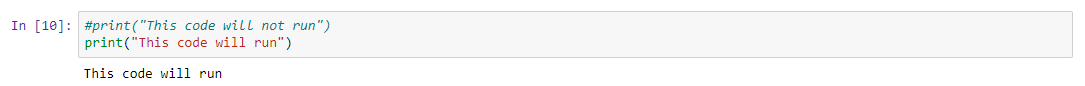In the above example, I show that the commented line will not print but the line under the commented line will print.

Errors
Uh oh, I was running my code and encountered an error.

`print(Hello World)`

When something is wrong with your code, Python will let you know through an Error message. These Errror messages are handy, and Python will do its best to direct you to the specific place in a line of code where the error occurred.
Shown above, we see SyntaxError: invalid syntax. What went wrong?
Something handy to do in these cases is to copy and paste the error into google. There are limitless resources on how to fix these errors and it is likely someone has encountered the same Error that you are trying to fix.

Oh, I think I see the problem. We forgot to define Hello World as a string. Let’s make Hello World a string by adding quotation marks.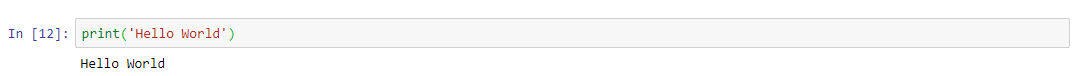We fixed our Error by adding quotation marks to Hello World, defining it as a string.

Variables
Alright, so we learned a little about data types. Let’s continue to explore them through the use of variables.

`myvar = 23myvar2 = 'Hello World'`

In the code snippet shown above, we see that I have declared two new variables, myvar and myvar2, through the use of the equal sign ‘=’. Whenever I use myvar or myvar2, it will recall the information that I have stored as these variables.

`myvar`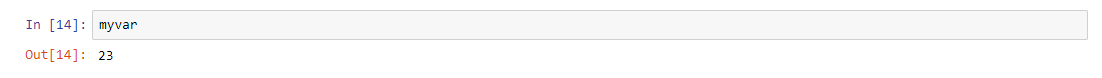Now whenever we use myvar in our code after declaring it is as a variable, it will recall that myvar = 23.

Variables must be defined (assigned to something through use of ‘=’) and the code must be executed. If you try to use a variable, but nothing is assigned, Python won’t know what to do and this NameError will show up:

Variables are useful because they allow us to recall information so that it does not need to be manually entered each time we want to use it. Furthermore, sometimes we want to set huge amounts of data as variables and therefore they are efficient. They also aid in creating generalizable algorithms and functions. We can try some of the methods that we learned on our variables.

Notice how, on the last code snippet (21), it only showed the type for myvar2. That is because Python only spits out the last line of code in the cell. If we want to see both types using only one cell, we must print each type:

`print(type(myvar))print(type(myvar2))`

Why would we want to include two methods in one cell? Because it is efficient. When you become an expert you may want to run hundreds of print methods at different sections in your functions.

Arithmetic Operators
Python can perform mathematical operations. We can perform addition, subtraction, multiplication and division. Let’s give it a try using variables:

`name1 = 'Spongebob'name2 = 'Squarepants'`

So, as we can see, we can add (or concatenate) strings together. If we simply add our variables, it jams the strings together (shown in Out). So, in this case, I added each variable with a string space (“ ”) in between them (In). I really just wanted to show you that the operators aren’t necessarily limited to integers or floats. Below is the more intuitive use of these operators (notice the use of comments at the top of each cell to show which operator is being used).

We can also set variables to other variables or as functions of other variables.

`Variable1 = 5Variable2 = 2Result = Variable1 + Variable2print(Result)`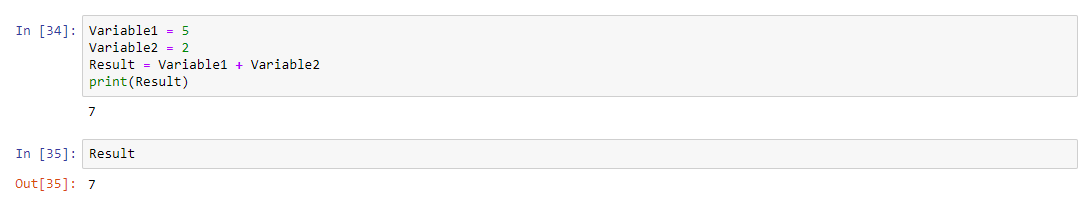We set Variable1 = 5 and Variable2 = 2. Then we create a new variable, Result, which is the sum of Variable1 and Variable2.

To go a little more in-depth, let’s use our print method but, this time, let’s mix and match integers and strings by using variables. To do this, we will use print() but we will need to separate each different data type by a comma.

`Variable1 = 8Variable2 = 9Result = Variable1 + Variable2print(Variable1,'+',Variable2,'is',Result)`

Let me introduce a different way of writing the same code using variables. We will use %s which is a string literal, and what it does is that it specifies where in the string you would like to insert a variable. Each time you input a string literal, you will need a variable to match and in the order of which you would like them to appear. You will need to separate the string from the variables with a % and parentheses surrounding your variables. I realize this probably doesn’t make much sense and it is not easy to explain
A picture is worth a thousand words:

`print("%s + %s is %s" % (Variable1, Variable2, Result))`

Inputs
For the last part of the lesson, let’s expand on what we learned about variables by learning about the input() method. The input() statement allows us to get input from a user. We can set this input to a variable. By writing a string in the parentheses of the input() method, we can elaborate on what kind of input we are looking for. Let’s write some simple code that asks for a name and then, when given the name, returns a courteous message using that name.

`Name = input("Hello, what is your name?: ")print('Hello',Name,',it is nice to meet you!')`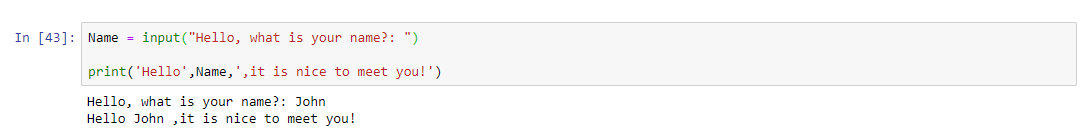With this code, the user will need to type their name because of the input() method. The courteous message will then be shown to that user, using their input.

Let’s do one last example.

`Variable1 = int(input('Give me a number '))Variable2 = int(input('Give me a second number '))Result = Variable1 + Variable2print('When I add your numbers, I get',Result)`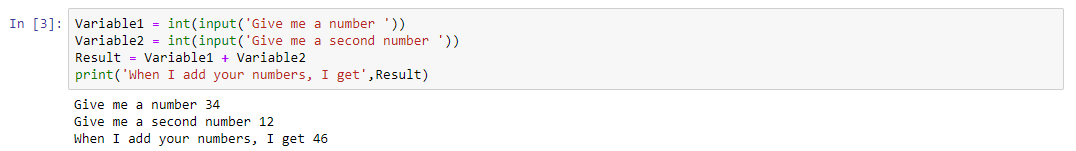The user will need to input 2 numbers. After getting user input, the user will be shown the sum of their inputs.

You’ll notice that I used an int() method to wrap the inputs. That is because inputs are strings unless specified otherwise. By wrapping the input() methods with an int() method, I’m setting the input to be an integer so that the numbers can be added together. If I had not wrapped the input with the int() method, here is what would have happened: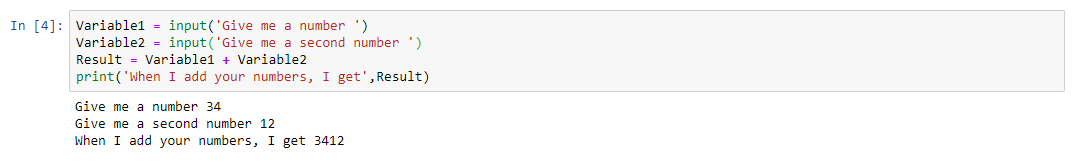This line is much like the SpongebobSquarepants example above. Because the inputs were not wrapped with the int() method, the inputs were taken as strings instead of integers and were improperly jammed together which does not make sense in this context.

Parting Notes
If you have issues with your notebook freezing, you may need to shut it down and start it back up again. This could happen especially when messing with Inputs. Be mindful of your data types. Also, be mindful about the inclusion of parentheses and quotation marks: these are the two most common sources of errors for beginners.

If you have had fun learning in this basic guide for Python, be sure to follow me so that you can catch the next post. I hope you have enjoyed this guide and I also hope that it has helped you get an idea of how to begin writing code in Python. Don’t be afraid to play around with what you’ve seen today, I believe that it is the best way to learn to code.

The next part of this lesson can be found here:

https://johnkundycki.medium.com/how-to-python-the-basics-ii-9de73c82a311

--

--